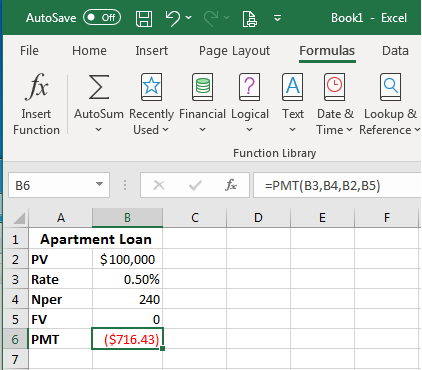# 8.16: Financial Functions and Formulas

$$\newcommand{\vecs}{\overset { \rightharpoonup} {\mathbf{#1}} }$$ $$\newcommand{\vecd}{\overset{-\!-\!\rightharpoonup}{\vphantom{a}\smash {#1}}}$$$$\newcommand{\id}{\mathrm{id}}$$ $$\newcommand{\Span}{\mathrm{span}}$$ $$\newcommand{\kernel}{\mathrm{null}\,}$$ $$\newcommand{\range}{\mathrm{range}\,}$$ $$\newcommand{\RealPart}{\mathrm{Re}}$$ $$\newcommand{\ImaginaryPart}{\mathrm{Im}}$$ $$\newcommand{\Argument}{\mathrm{Arg}}$$ $$\newcommand{\norm}{\| #1 \|}$$ $$\newcommand{\inner}{\langle #1, #2 \rangle}$$ $$\newcommand{\Span}{\mathrm{span}}$$ $$\newcommand{\id}{\mathrm{id}}$$ $$\newcommand{\Span}{\mathrm{span}}$$ $$\newcommand{\kernel}{\mathrm{null}\,}$$ $$\newcommand{\range}{\mathrm{range}\,}$$ $$\newcommand{\RealPart}{\mathrm{Re}}$$ $$\newcommand{\ImaginaryPart}{\mathrm{Im}}$$ $$\newcommand{\Argument}{\mathrm{Arg}}$$ $$\newcommand{\norm}{\| #1 \|}$$ $$\newcommand{\inner}{\langle #1, #2 \rangle}$$ $$\newcommand{\Span}{\mathrm{span}}$$$$\newcommand{\AA}{\unicode[.8,0]{x212B}}$$

Learning Objectives

• Use financial functions and formulas

Excel provides specific formulas and functions to assist with financial calculations. We will cover the top five most often used financial functions. The following scenario will inform the next example of financial functions.

A person secured a loan from a bank to purchase an apartment at an annual interest rate of 6%, over 20 years, with monthly payments. The loan has a present value (PV) of $100,000 (amount of new loan) and a future value (Fv) of 0 (because the goal of the loan is to have it completely paid off at the end of the time period). For the monthly payments use 6%/12 = 0.5% for Rate, and 20*12 = 240 for Nper (total number of payment periods). If only annual payments were made, then it we would use 6% for the Rate and 20 for Nper. ## Payment (PMT) Payment terms for a loan or investment. The Excel formula for it is =PMT(rate,nper,pv,[fv],[type]). This assumes that payments are made on a consistent basis. Follow these steps to find the monthly payment amount for this loan: 1. Enter all the information into a table. 2. Using the Formulas tab, Financial button, scroll until you find PMT in the drop-down menu.3. A dialog window asking for the inputs opens. Click on the cells matching the correct information for that field and the data will be entered into the formula automatically. Using the cell location instead of typing in the straight data, means the formula will automatically update if new data is entered into those cells. For the last two areas (Fv,Type) for loans, Fv can be omitted (or enter 0) and Type can also be left empty. Type left empty assumes that payments come due at the end of the period.4. After the calculation, the monthly loan payment is$716.43. The figure is red because it is a debt paid against the total loan. (If you wish to have it not show as a red/negative number, type in a minus sign before the B2 Rate (-B2) and the PMT will show as a black, positive number.) The second screenshot shows the PMT formula and the corresponding cells associated with the formula. To see the formula correspondence, double clicking on the cell. This correspondence association works with any formulas used.5. With the formula established, when you change the rate, present value or nper, you can see the change in monthly payment it will take to pay off the loan.

## Number of Periods (NPER)

Number of periods per loan or investment. The Excel formula for this is =NPER(rate,pmt,pv,[fv],[type]).

Follow these steps to find the number of periods for this loan:

1. Enter all the information into a table.
2. Using the Formulas tab, Financial button, scroll until you find NPER in the drop-down menu.
3. A dialog window asking for the inputs opens. Enter the corresponding cell locations.4. The Nper is 240 months (20 years of monthly payments). The second screenshot shows the NPER formula and the corresponding cells associated with the formula. Double click on the cell to see its corresponding cells.
5. With the formula established, changing the monthly payment amount shows the change in the number of periods needed to pay off the loan.## Rate (RATE)

The interest rate for a loan or a rate of return required to attain a specific amount on an investment over a period of time. The Excel formula for this is =RATE(nper,pmt,pv,[fv],[type],[guess]). The “guess” for our purposes can be left out for this scenario.

Follow these steps to find the interest rate for this loan:

1. Enter all the information into a table.
2. Using the Formulas tab > Financial button > Scroll until you find RATE in the drop-down menu.
3. Dialog window opens > Enter the corresponding cell locations.
4. The Rate is 0.50%. The second screenshot shows the Rate formula and the corresponding cells associated with the formula. Double click on the cell to see its corresponding cells.

## Present Value (PV)

Present value of a loan or investment based on a constant interest rate (like a mortgage or loan). The Excel formula for this is =PV(rate,nper,pmt,[fv],[type]).

Let’s change the scenario a little to find a PV for a different loan. If you know that the monthly payments are $952.69, the interest rate is 6%, and the life of the loan is 20 years, what is the present value of that loan? Follow the same steps to insert the formulas like before to find the present value for this loan. After filling in the values for the above scenario the PV for this loan is$120,999.66 or if rounded up $121,000. ## Future Value (FV) Future value of an investment assuming constant, periodic payments with a constant interest rate. The Excel formula for this is =FV(rate,nper,pmt,[pv],[type]). Let’s change the scenario again and find FV for a different loan’s time frame. If you know that the monthly payments are$895.54, the interest rate is 5%, and the life of the loan is 20 years, is the future value of that loan payed down to 0 in that 20-year time frame?

Follow the same steps to insert the formulas like before only using the FV function to figure this out.

The answer is that, yes, the future value of the loan comes to zero in the timeframe.

Now you know how to use these five financial functions and formulas in Excel.